### Transfer learning for time series classification

This is the companion web page for our paper titled "Transfer learning for time series classification".

This paper has been accepted at IEEE Big Data 2018 conference.

### The source code

The software is developed using Python 3.5. We trained the models on a cluster of more than 60 GPUs. You will need the UCR archive to re-run the experiments of the paper. The source code can be downloaded here upon the acceptance of the paper.

To run the code you will also need to download seperatly and install the following dependencies:

### Raw results

You can download here the accuracy variation matrix which corresponds to the raw results of figure 9 in the paper.

You can download here the result of the applying nearest neighbor algorithm on the inter-datasets similarity matrix. You will find for each dataset in the archive, the 84 most similar datasets.

The steps for computing the similarity matrix are presented in Algorithm 1 in our paper.

### Pre-trained and fine-tuned models

You can download part-1 and part-2, the compressed fine-tuned models (with transfer learning). There are two parts that should be in the same folder but for one-file-size server limitation we divided them into two archives.

Each archive contains a folder for each source dataset. In each of these folders you have a folder for each of the remaining 84 target datasets. In each of these folders, you can find the corresponding architecture (architecture.txt file) in a json format which can be loaded using the function model_from_json in Keras. Then using the function load_weights in Keras, you can load the corresponding weights (best_model_weights.hdf5 file) which have been fine-tuned.

The archive contains a folder for each dataset. In each of these folders you will find the model in a HDF5 format (best_model.hdf5 file). This file contains both the architecture and the pre-trained weights which can be loaded using the function load_model in Keras.

A full tutorial on how to load models, weights and architectures using the Keras library is available here.

You will also need to compile the model with the Adam optimizer and set the hyper-parameters to be equal to the one we mentioned in our paper. The code can be find here.

### Genrealization with and without the transfer learning

You can find here the evolution of the model's loss during the training.

The archive contains a folder for each source dataset. In each of these folders you have a folder for each of the remaining 84 target datasets (where you can find the plot in a pdf format).

These plots are similar to figure 1 in the paper.

The following are three examples: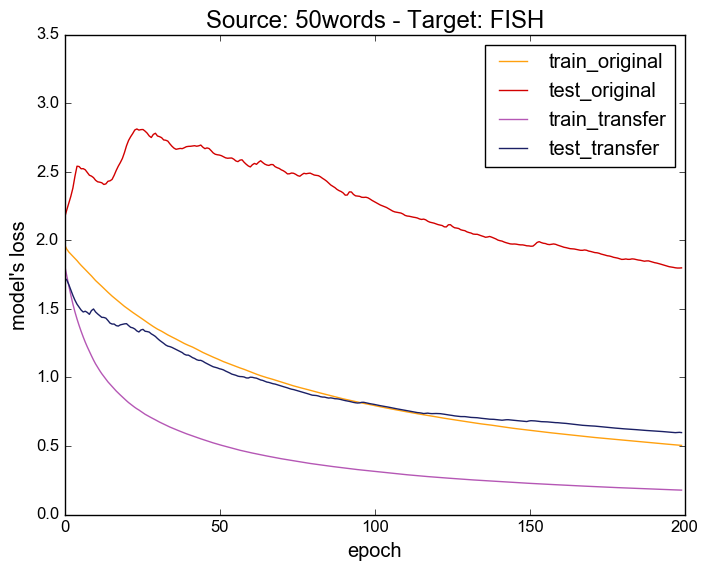Source: 50words - Target: FISH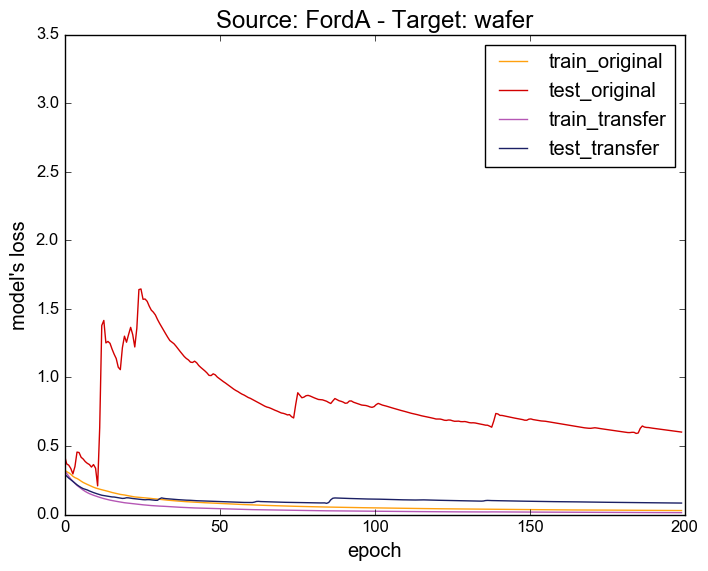Source: FordA - Target: wafer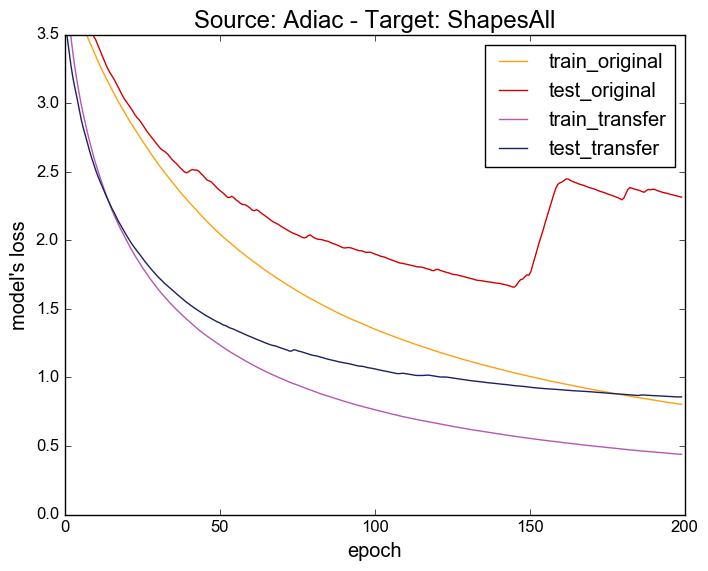### Model's accuracy with respect to the source dataset's similarity

You can find here the plots for each target dataset in the UCR archive while varying the source dataset.

These plots are similar to figures 6,7 and 8 in the paper, but due to space limitations we have chosen three interesting case studies.

The following are another three examples: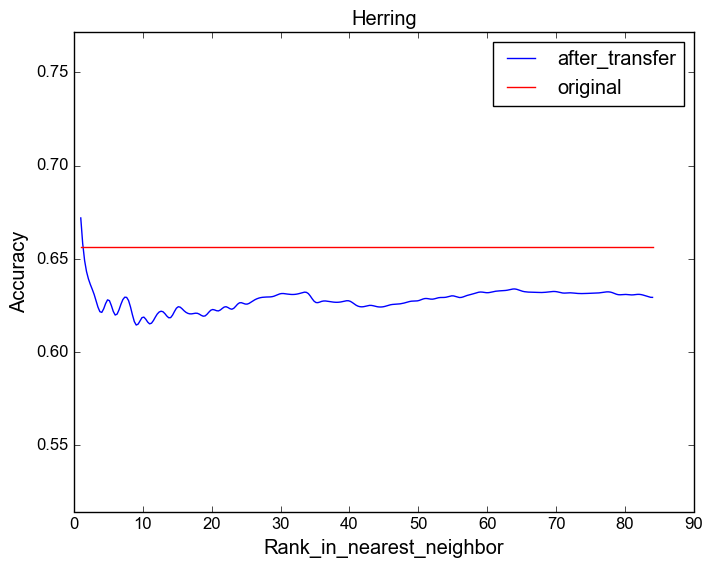Target dataset: Herring.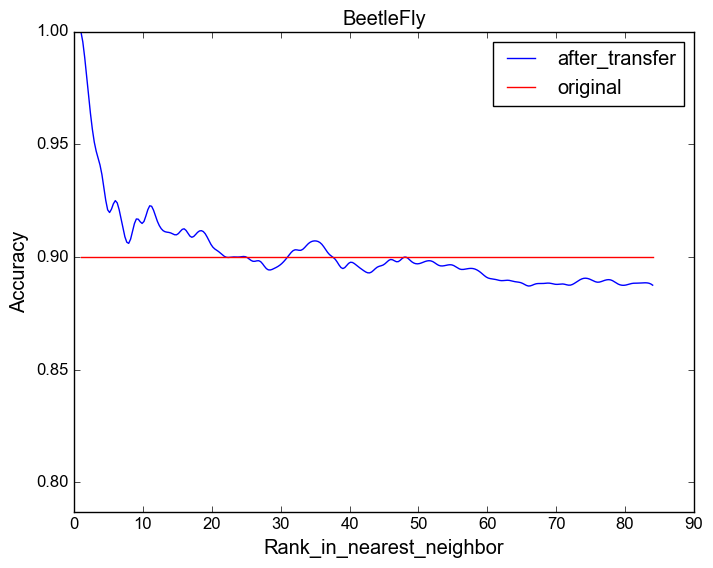Target dataset: BeetleFly.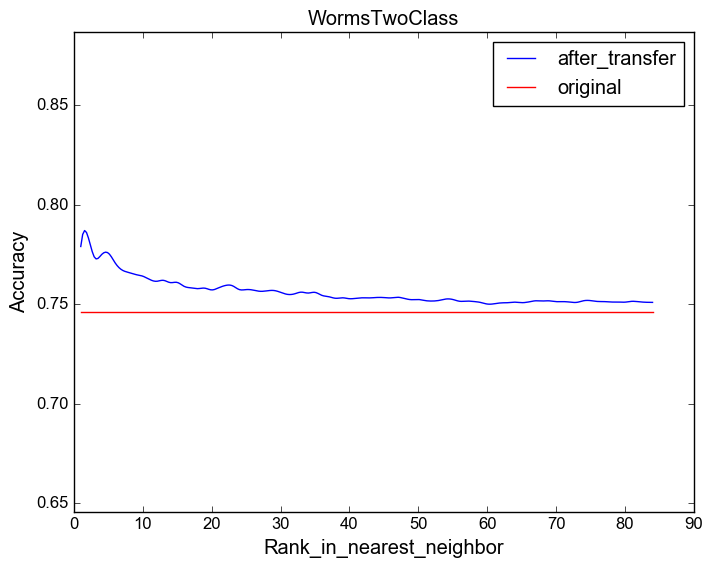Target dataset: WormsTwoClass.

Last update : November 2018